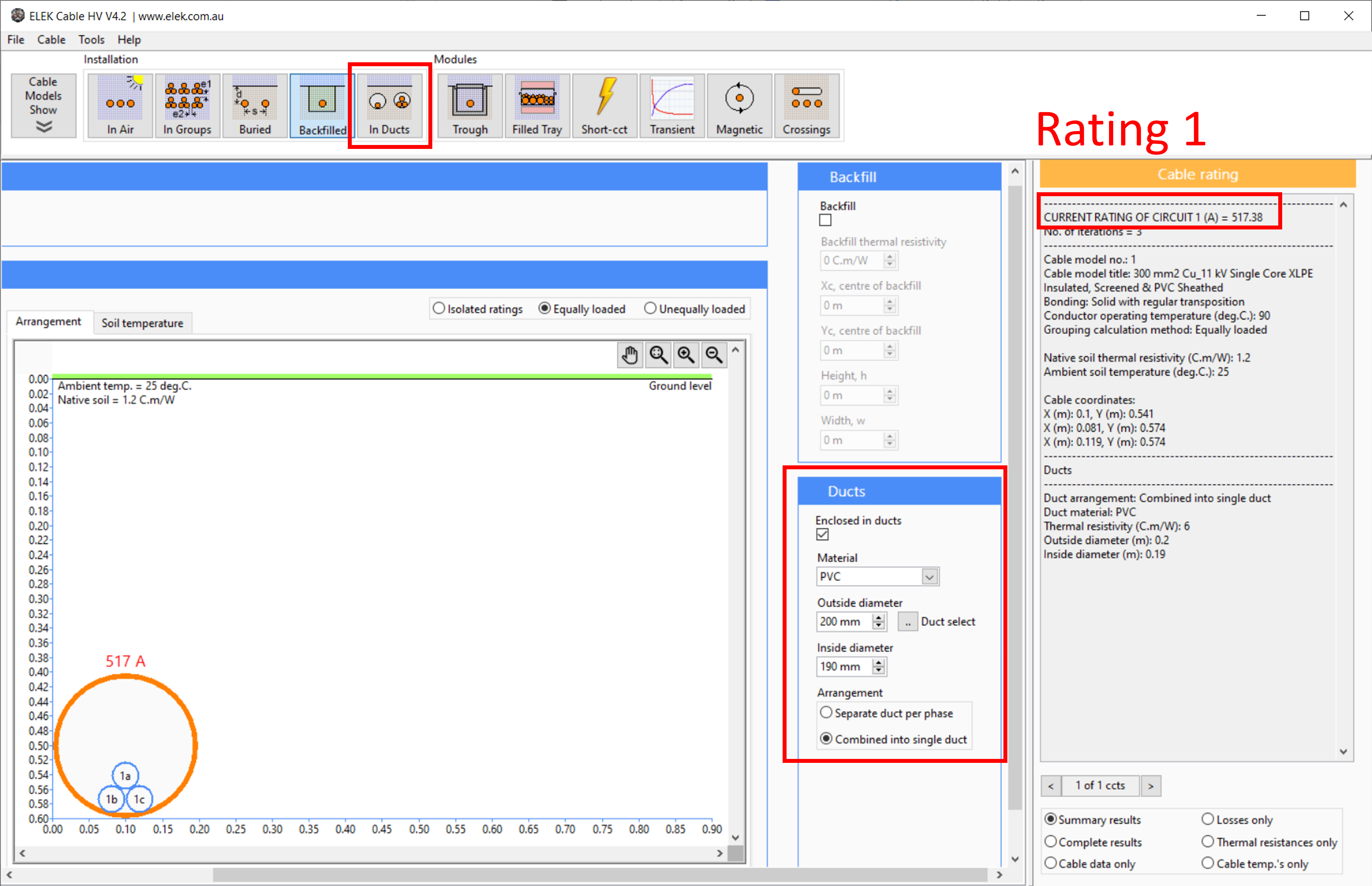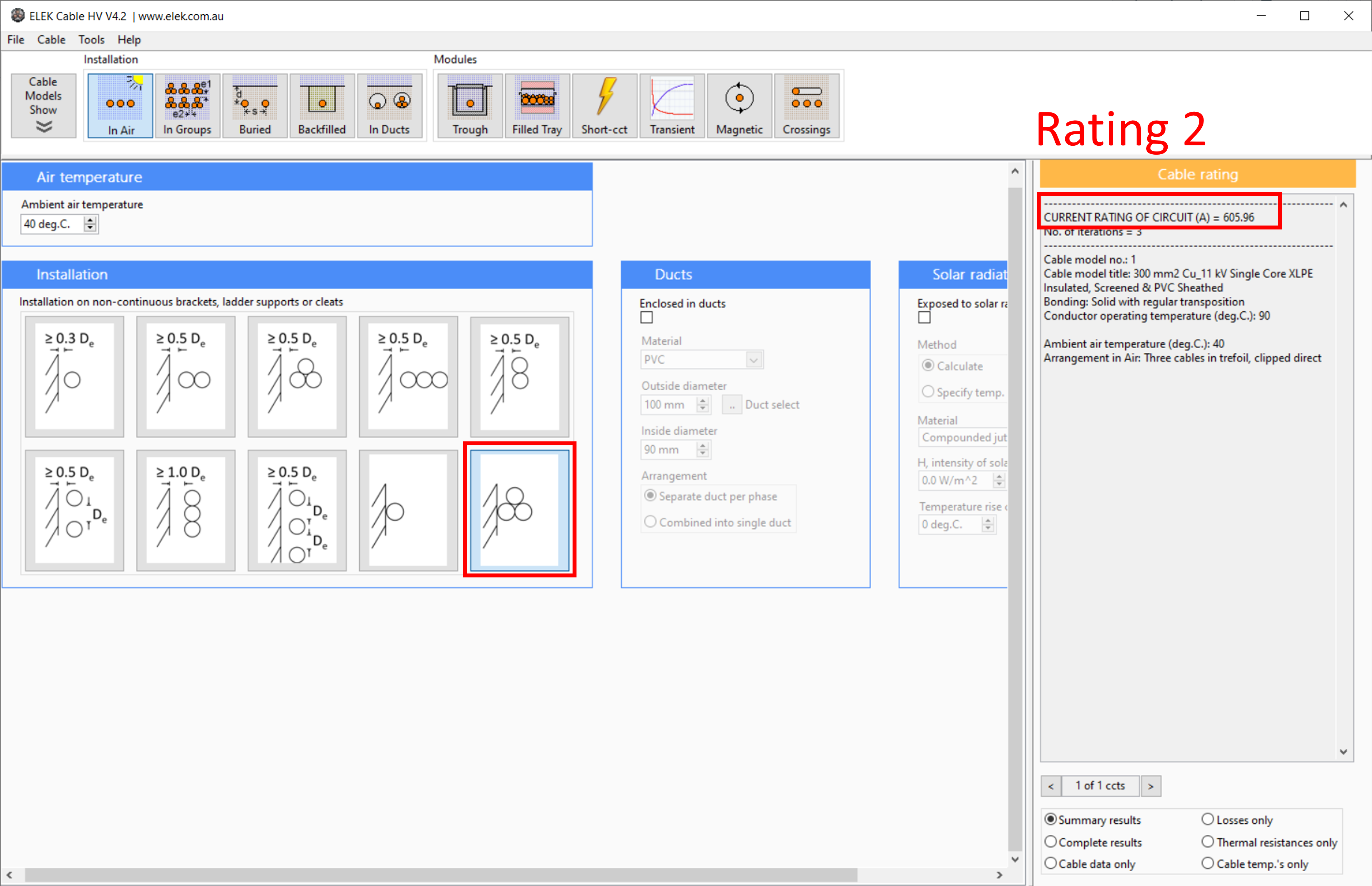This problem is not covered directly by the IEC Standards (i.e. they only cover the rating for 1 circuit in a duct).  To solve this problem using the IEC equations is a multi-step process using Cable HV Software.

This method has the following assumptions:

• Cables are the same type.### Example calculation - 3 circuits installed in the same buried duct

In this example we will be calculating the current rating for multiple circuits (3 circuits) installed inside the same buried duct.

Step 1: Calculate the current rating for a single circuit (3 cables) buried in the same duct.

Make sure the duct size being modelled is the same duct size which will house the multiple circuits.

Rating 1 in this example = 517.38 A

This is the current rating for a single circuit installed in the same duct.

Next we will derive a custom derating factor for multiple circuits.Step 2: Calculate the current rating for a single circuit installed in air.

Use the installation method called "Three cables in trefoil, clipped direct" (as shown) to include that while installed inside a duct the cables will be touching the inside walls of the duct.

Rating 2 in this example = 605.96 A

This is the current rating for a single circuit installed in air.Step 3: Calculate the current rating for multiple circuit installed in air In Groups.

Use the installation method called "Side by side_3 trefoils" (as shown) and make sure the Clearance, e = 0 m to include the thermal proximity effect (mutual heating between the circuits in air).

Rating 3 in this example = 578.8 A

This is the current rating for 3 circuits installed (touching) in air in a group as they would be inside the duct together.Final step: Calculate the derating factor for the groups in air and apply it to the buried case rating.

Use the installation method called "Side by side_3 trefoils" (as shown) and make sure the Clearance, e = 0 m to include the thermal proximity effect (mutual heating between the circuits in air).

This is the current rating for 3 circuits installed (touching) in air in a group as they would be inside the duct together.

The final rating for multiple circuits installed in one duct = (Rating 3/ Rating 2) x Rating 1

Where Rating 3 / Rating 2 (578.8 A/605.96 A=0.9552) is the derating factor to be applied to Rating 1.

Final current rating of a circuit installed inside the same duct (3 circuits in total) = 494.94 A